# KSEEB SSLC Class 10 Maths Solutions Chapter 9 Polynomials Ex 9.3

KSEEB SSLC Class 10 Maths Solutions Chapter 9 Polynomials Ex 9.3 are part of KSEEB SSLC Class 10 Maths Solutions. Here we have given Karnataka SSLC Class 10 Maths Solutions Chapter 9 Polynomials Exercise 9.3.

## Karnataka SSLC Class 10 Maths Solutions Chapter 9 Polynomials Exercise 9.3

Question 1.
Divide the polynomial p(x) by the polynomial g(x) and find the quotient and remainder in each of the : following.
(i) p(x) = x3 – 3x2 + 5x – 3; g(x) = x2 – 2
(ii) p(x) = x4 – 3x2 + 4x + 5; g(x) = x2 + 1 – x
(iii) p(x) = x4 – 5x + 6; g(x) = 2 – x2.
Solution:
(i) p(x) = x3 – 3x2 + 5x – 3
g(x) =x2 – 2
q(x) = ?
r(x) = ?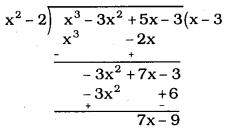∴ Quotient, q(x) = (x – 3)
Remainder, r(x) = (7x – 9)

(ii) p(x) = x4 – 3x2 + 4x + 5
g(x) = x2 + 1 – x
q(x) = ?
r(x) = ?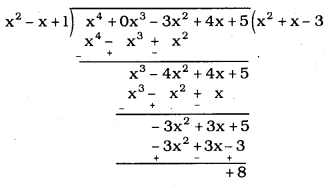∴ Quotient, q(x) = x2 + x – 3
Remainder, r(x) = 8

(iii) p(x) = x4 – 5x + 6
g(x) = 2 – x2
q(x) = ?
r(x) = ?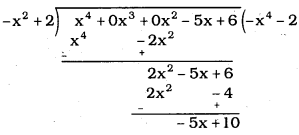∴ Quotient, q(x) = -x2 – 2
Remainder, r(x) = -5x + 10

Question 2.
Check whether the first polynomial is a factor of the second polynomial by dividing the second polynomial by the first polynomial :
(i) t2 – 3; 2t4 + 3t3 – 2t2 – 9t – 12
(ii) x2 + 3x + 1; 3x4 + 5x3 – 7x2 + 2x + 2
(iii) x3 – 3x + 1; x5 – 4x3 + x2 + 3x + 1
Solution:
(i) t2 – 3; 2t4 + 3t3 – 2t2 – 9t – 12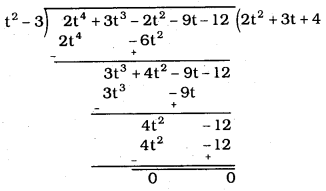∴ t2 – 3 is the factor of 2t4 + 3t3 – 2t2 – 9t – 12 because remainder is zeroes

(ii) x2 + 3x + 1; 3x4 + 5x3 – 7x2 + 2x + 2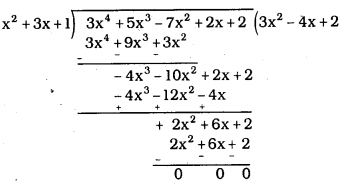Here remainder is zero
∴ x2 + 3x + 1 is the factor of 3x4 + 5x3 – 7x2 + 2x + 2

(iii) x3 – 3x + 1; x5 – 4x3 + x2 + 3x + 1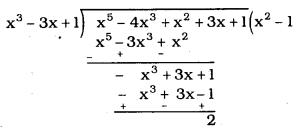Here remainder r(x) ≠ 0 ∵ 2 ≠ 0
∴ x3 – 3x + 1 is not the factor of x5 – 4x3 + x2 + 3x + 1

Question 3.
Obtain all other zeroes of 3x4 + 6x3 – 2x2 – 10x – 5, if two of its zeroes are $$\sqrt{\frac{5}{3}}$$ and $$-\sqrt{\frac{5}{3}}$$
Solution:
Roots of expressions are $$\sqrt{\frac{5}{3}}$$ and $$-\sqrt{\frac{5}{3}}$$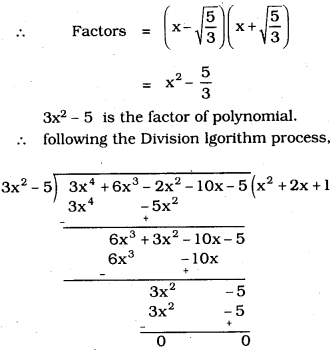∴ In q(x) = x2 + 2x + 1, there are two more roots.
x2 + 2x + 1 = (x + 1)2
other roots are: x = -1, -1

Question 4.
On dividing x3 – 3x2 + x + 2 by a polynomial g(x), the quotient and remainder were x – 2 and -2x + 4, respectively. Find g(x).
Solution:
x3 – 3x2 + x + 2 = g(x) × (x – 2) + (-2x + 4)
g(x) × (x – 2) = x3 – 3x2 + x + 2 – (-2x + 4)
= x3 – 3x2 + x + 2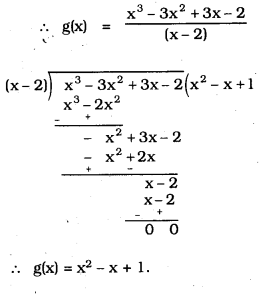Question 5.
Give examples of polynomials p(x), g(x), q(x) and r(x), which satisfy the division algorithm and
(i) deg p(x) = deg q(x)
(ii) deg q(x) = deg r(x)
(iii) deg r(x) = 0
Solution:
i) P(x) = 2x2 – 2x + 14, g(x) = 2,
q(x) = x2 – x + 7 and r(x) = 0
Verification
P(x) = g(x) q(x) + r(x)
2x2 – 2x + 14 = 2(x2 – x + 7) + 0
= 2x2 – 2x + 14
∴ LHS = RHS

ii) P(x) = x3 + x2 + x + 1, g(x) = x2 – 1, q(x) = x + 1 and r(x) = 2x + 2
Verification
P(x) = q(x) g(x) + r(x)
x3 + x2 + x + 1 =(x+ 1) (x2 – 1) + 2x + 2
= x3 – x + x2 – 1 + 2x + 2
= x3 + x2 + x + 1
LHS = RHS

iii) P(x) = x3 + 2x2 – x + 2, g(x) = x2 – 1, q(x) = x + 2 and r(x) = 4
p(x) = g(x) q(x) + r(x)
x3 + 2x2 – x + 2 = (x2 – 1) (x + 2) + 4
= x3 + 2x2 – x – 2 + 4
= x3 + 2x2 – x + 2
LHS = RHS

We hope the given KSEEB SSLC Class 10 Maths Solutions Chapter 9 Polynomials Ex 9.3 will help you. If you have any query regarding Karnataka SSLC Class 10 Maths Solutions Chapter 9 Polynomials Exercise 9.3, drop a comment below and we will get back to you at the earliest.